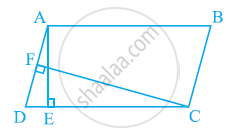Share

# In the given figure, ABCD is parallelogram, AE ⊥ DC and CF ⊥ AD. If AB = 16 cm, AE = 8 cm and CF = 10 cm, find AD. - CBSE Class 9 - Mathematics

ConceptParallelograms on the Same Base and Between the Same Parallels

#### Question

In the given figure, ABCD is parallelogram, AE ⊥ DC and CF ⊥ AD. If AB = 16 cm, AE = 8 cm and CF = 10 cm, find AD.#### Solution

In parallelogram ABCD, CD = AB = 16 cm

[Opposite sides of a parallelogram are equal]

We know that

Area of a parallelogram = Base × Corresponding altitude

Area of parallelogram ABCD = CD × AE = AD × CF

16 cm × 8 cm = AD × 10 cm

AD = (16xx8)/10cm=12.8cm

Thus, the length of AD is 12.8 cm.

Is there an error in this question or solution?

#### APPEARS IN

NCERT Solution for Mathematics Class 9 (2018 to Current)
Chapter 9: Areas of Parallelograms and Triangles
Ex. 9.20 | Q: 1 | Page no. 159

#### Video TutorialsVIEW ALL 

Solution In the given figure, ABCD is parallelogram, AE ⊥ DC and CF ⊥ AD. If AB = 16 cm, AE = 8 cm and CF = 10 cm, find AD. Concept: Parallelograms on the Same Base and Between the Same Parallels.
S